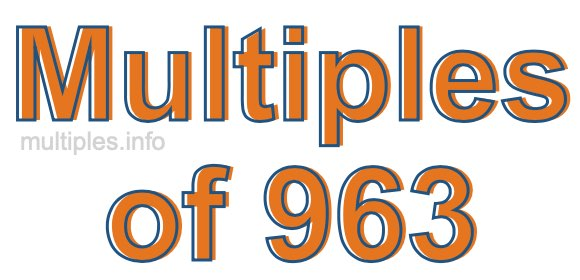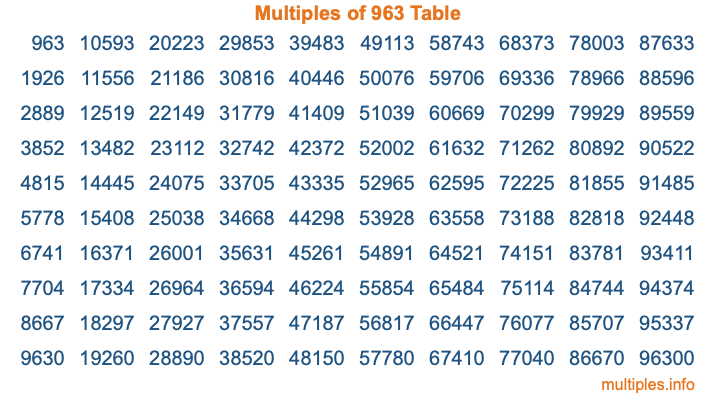Multiples of 963Welcome to the Multiples of 963 page. Here we will first teach you everything you will ever need to know about the multiples of 963, and then give you a study guide summary of everything we taught you to make sure you remember it all. Use this page to look up facts and learn information about the multiples of 963. This page will make you a multiples of nine hundred sixty-three expert!

Definition of Multiples of 963
Multiples of 963 are all the numbers that when divided by 963 equal an integer. Each of the multiples of 963 are called a multiple. A multiple of 963 is created by multiplying 963 by an integer.

Therefore, to create a list of multiples of 963, you start with 1 multiplied by 963, then 2 multiplied by 963, then 3 multiplied by 963, and so on for as long as you want. Thus, the list of the first five multiples of 963 is 963, 1926, 2889, 3852, and 4815. To see a larger list of multiples of 963, see the printable image of Multiples of 963 further down on this page. We also have a category where you can choose any nth multiple of 963.

Multiples of 963 Checker
The Multiples of 963 Checker below checks to see if any number of your choice is a multiple of 963. In other words, it checks to see if there is any number (integer) that when multiplied by 963 will equal your number. To do that, we divide your number by 963. If the the quotient is an integer, then your number is a multiple of 963.

Is  a multiple of 963?

Least Common Multiple of 963 and ...
A Least Common Multiple (LCM) is the lowest multiple that two or more numbers have in common. This is also called the smallest common multiple or lowest common multiple and is useful to know when you are adding our subtracting fractions. Enter one or more numbers below (963 is already entered) to find the LCM.

Check out our LCM Calculator if you need more details about the Least Common Multiple or if you need the LCM for different numbers for adding and subtraction fractions.

nth Multiple of 963
As we stated above, 963 is the first multiple of 963, 1926 is the second multiple of 963, 2889 is the third multiple of 963, and so on. Enter a number below to find the nth multiple of 963.

th multiple of 963

Multiples of 963 vs Factors of 963
963 is a multiple of 963 and a factor of 963, but that is where the similarities end. All postive multiples of 963 are 963 or greater than 963. All positive factors of 963 are 963 or less than 963.

Below is the beginning list of multiples of 963 and the factors of 963 so you can compare:

Multiples of 963: 963, 1926, 2889, 3852, 4815, etc.

Factors of 963: 1, 3, 9, 107, 321, 963

As you can see, the multiples of 963 are all the numbers that you can divide by 963 to get a whole number. The factors of 963, on the other hand, are all the whole numbers that you can multiply by another whole number to get 963.

It's also interesting to note that if a number (x) is a factor of 963, then 963 will also be a multiple of that number (x).

Multiples of 963 vs Divisors of 963
The divisors of 963 are all the integers that 963 can be divided by evenly. Below is a list of the divisors of 963.

Divisors of 963: 1, 3, 9, 107, 321, 963

The interesting thing to note here is that if you take any multiple of 963 and divide it by a divisor of 963, you will see that the quotient is an integer.

Multiples of 963 Table
Below is an image of the first 100 multiples of 963 in a table. The table is in chronological order, column by column. The first column has the first ten multiples of 963, the second column has the next ten multiples of 963, and so on.The Multiples of 963 Table is also referred to as the 963 Times Table or Times Table of 963. You are welcome to print out our table for your studies.

Negative Multiples of 963
Although not often discussed or needed in math, it is worth mentioning that you can make a list of negative multiples of 963 by multiplying 963 by -1, then by -2, then by -3, and so on, to get the following list of negative multiples of 963:

-963, -1926, -2889, -3852, -4815, etc.

Multiples of 963 Summary
Below is a summary of important Multiples of 963 facts that we have discussed on this page. To retain the knowledge on this page, we recommend that you read through the summary and explain to yourself or a study partner why they hold true.

There are an infinite number of multiples of 963.

A multiple of 963 divided by 963 will equal a whole number.

963 divided by a factor of 963 equals a divisor of 963.

The nth multiple of 963 is n times 963.

The largest factor of 963 is equal to the first positive multiple of 963.

963 is a multiple of every factor of 963.

963 is a multiple of 963.

A multiple of 963 divided by a divisor of 963 equals an integer.

963 divided by a divisor of 963 equals a factor of 963.

Any integer times 963 will equal a multiple of 963.

Multiples of a Number
Here you can get the multiples of another number, all with the same attention to detail as we did for multiples of 963 on this page.

Multiples of
Multiples of 964
Did you find our page about multiples of nine hundred sixty-three educational? Do you want more knowledge? Check out the multiples of the next number on our list!